##### Trigonometry Workbook For DummiesThree basic trigonometry identities involve the sums of angles; the functions involved in these identities are sine, cosine, and tangent. You can also adapt these three basic angle-sum identities for the other three functions (cosecant, secant, and cotangent) by using the reciprocal identities.

When confronted with a reciprocal identity, you change the expression to one of the three basic functions, do the necessary work, and then use the reciprocal identity to change the answer back in terms of the function you began with.

You can use the angle-sum identities to find the function values of any angles, but the following examples just show the most convenient combinations — ones with exact values that you can easily fill into the formulas. Suppose, for example, that you want to find the exact value of the sine of 75 degrees. To minimize fuss, you can use the sum of 30 degrees and 45 degrees and the appropriate identity; this uses angles whose functions have nice, exact values.

The angle-sum identities find the function value for the sum of angle and angle :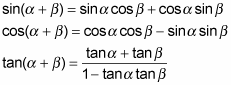Using the identity for the sine of a sum, find the sine of 75 degrees:

1. Determine two angles whose sum is 75 for which you know the values for both sine and cosine.

Choose 30 + 45, not 50 + 25 or 70 + 5, because sticking to the more-common angles that have nice, exact values to use in the formula is your best bet.

2. Input the angle measures into the identity.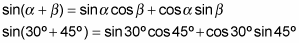3. Replace the functions of the angles with their values and simplify.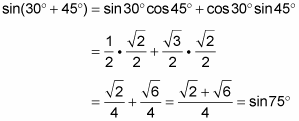Sometimes, you have more than one choice for the sum. In this example, find the cosine of 120 degrees by using the identity for the cosine of a sum.

1. Determine two angles whose sum is 120.

Choosing among the most convenient angles, you can use either 90 + 30 or 60 + 60. This example uses 90 + 30, because the sine of a 90-degree angle is 1, and the cosine is equal to 0. Both of those numbers are very nice to have in a computation because they keep it simple.

2. Input the values into the identity.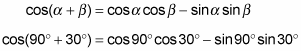3. Replace the functions with their values and simplify.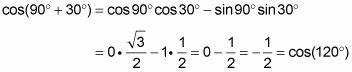4. Determine two angles whose sum is 7π/12.

It may be easier to think of finding two numbers that add up to 7/12.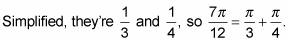5. Input the values into the identity.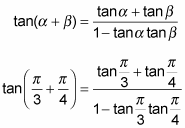6. Replace the functions with their values and simplify.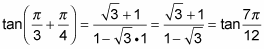The result in the last step doesn’t leave the answer in the nicest form. The denominator has two terms, and one of them is a radical. One way to make the answer look a bit better and more intelligible is to use a technique called rationalization.

To rationalize the numerator or denominator of a fraction, multiply both the numerator and denominator by the conjugate (same terms, opposite sign) of the part that you’re rationalizing. When you do so, you end up with the difference of two squares and get rid of the offending portion.

For the next example, you rationalize like so to get the radical out of the denominator: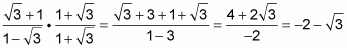The final answer is a bit nicer to understand and estimate.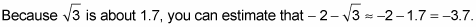This last example shows how to find csc 105° — using the reciprocal identity, along with the angle-sum identity.

1. Determine two angles whose sum is 105°.

Angles measuring 60 and 45 degrees have a sum of 105 degrees.

2. Choose an angle-sum identity.

Since the cosecant is the reciprocal of the sine, use the sine angle-sum to find the sine of 105 degrees.

3. Fill in the appropriate values.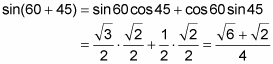4. Use the reciprocal identity to find csc 105°.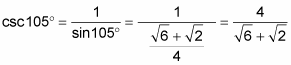Of course, you’ll want to rationalize this value and/or find a nice decimal equivalent.